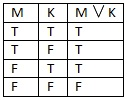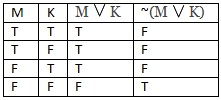# Lessons in Logic: Disjunction

Either or both, that’s the assumption
When that’s the case you’ve been served a disjunction

Another operator today, a disjunction is an “or” statement which requires only one or the other of the propositions to be true. Symbolized as, this operator allows us to recognize an important relation between propositions.

An example of a disjunction is “The cat is on the mat or the cat is in the kitchen”, whichwould be symbolized as “MK”. It can be true if the cat is on the mat, or it can be true if the cat is in the kitchen, but it will also be true if both of the propositions are true. Maybe the mat is in the kitchen, so the cat can both be on the mat and be in the kitchen. This can be seen in the truth table, and means that the only time a disjunction can be false is when both of the propositions is false. If the cat is outside in a tree, then it’s not true that the cat is on the mat, and it’s not true that the cat is in the kitchen, so it’s also not true that either the cat is on the mat or the cat is in the kitchen.

A disjunction interacts with other operators in much the same way that a conjunctiondoes. You can negate one or both parts of a disjunction like this, M~K, which means “The cat is on the mat or the cat is not in the kitchen”. Or you can negate the whole expression by bracketing it ~(MK), so that it’s not the case that either the cat is on the mat or the cat is in the kitchen. The truth table for this is a little more complex, but you can see that any time that MK would be true, ~(MK) is false.

There’s a different kind of disjunction which I won’t be using here but bears mentioning, and that’s the exclusive disjunction. The disjunction I’ve shown is true if one, the other, or both of the propositions are true, but for an exclusive disjunction the rule is “one or the other but not both”. It comes up a lot in math and programming logic, as I understand it. It’s unnecessary for us because we can illustrate the exclusion by using a conjunction, which would look like this: (MK)~(MK). Translated into English, this means “It’s the case that either the cat is on the mat or the cat is in the kitchen, and not the case that the cat is on the mat and the cat is in the kitchen”, so one or the other can be true, but not both.

That wraps up this week’s post! For updates on posts and other things philosophy related, like my page on Facebook! Next week’s lesson in logic introduces the most complex operator, the material conditional. Thanks for reading!